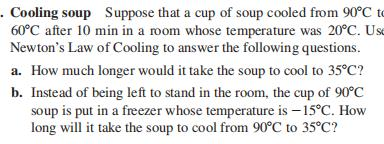### Still have math questions?

Trigonometry
QuestionCooling soup Suppose that a cup of soup cooled from $$90 ^ { \circ } C$$ t $$60 ^ { \circ } C$$ after $$10$$ min in a room whose temperature was $$20 ^ { \circ } C$$ . Ust Newton's Law of Cooling to answer the following questions.

a. How much longer would it take the soup to cool to $$35 ^ { \circ } C$$ ?

b. Instead of being left to stand in the room, the cup of $$90 ^ { \circ } C$$ soup is put in a freezer whose temperature is $$- 15 ^ { \circ } C$$ . How long will it take the soup to cool from $$90 ^ { \circ } C$$ to $$35 ^ { \circ } C$$ ?

$$\text { (a) } 17.5 min \text { (b) } 13.26 min$$